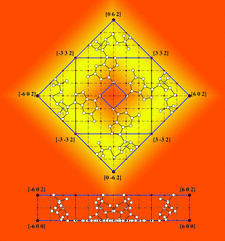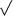issue contentsFOUNDATIONSADVANCES
ISSN: 2053-2733

September 2007 issueCover illustration: The molecular form of the guanosine-5'-phosphate tetramer [Zimmerman (1976). J. Mol. Biol. 106, 663-672] has vertices at points of a cubic lattice with parameter a defined by the distance of O6 from the centre. The height is H = 2a and the basis a square scaled by a factor 6 from the central hole. Another square, scaled by 32 and turned by 45°, separates phosphates and bases. The indices of the molecular forms are indicated. Courtesy of A. Janner.

research papers

Diffuse scattering from large-angle, thermally induced, orientational disorder in molecular crystals

A method is described of including large-angle librations in the scattering calculated from molecular solids. Results are presented for three sample materials.

Structural phase and amplitude measurement from distances in convergent-beam electron diffraction patterns

The first direct experimental measurements of structural phase and amplitude in a centrosymmetric crystal from distances in three-beam convergent-beam electron diffraction patterns are presented as a solution to the `phase problem'.

Random tilings of compact Euclidean 3-manifolds

Non-periodic deterministic and random tilings for the ten compact Euclidean 3-manifolds are introduced and their configurational entropies are computed.

A charge-flipping algorithm incorporating the tangent formula for solving difficult structures

An algorithm is described that incorporates the direct-methods tangent formula into the structure solution method of charge flipping and the subsequent avoidance of `uranium atom' solutions.

Scanning of magnetic space groups and the analysis of non-magnetic domain walls

Using scanning of magnetic space groups, the possible displacement of atoms at the center of non-magnetic domain walls is determined.

The introduction of structure types into the Inorganic Crystal Structure Database ICSD

The approach used and the progress made in the assignment of structure types to the crystal structures contained in the ICSD database are reported.

Three-periodic nets and tilings: natural tilings for nets

Rules for determining a unique tiling for a three-periodic net are presented

Can the interaction density be measured? The example of the non-standard amino acid sarcosine

Visualizing the crystal-field effect. A combined experimental and theoretical charge-density study on the non-standard amino acid sarcosine.

short communications

Idler energy dependence of nonlinear diffraction in X → X + EUV parametric down-conversion

The rocking curve of nonlinear X-ray diffraction in X → X + EUV parametric down-conversion was found to depend strongly on the idler energy.

book reviews

Personal X-ray reflections

Follow Acta Cryst. A